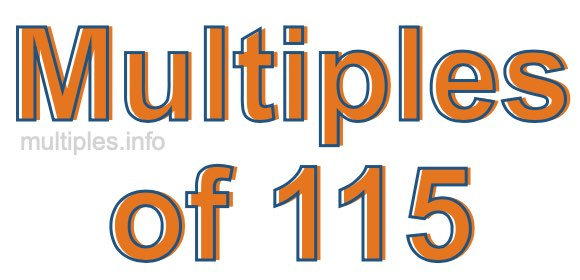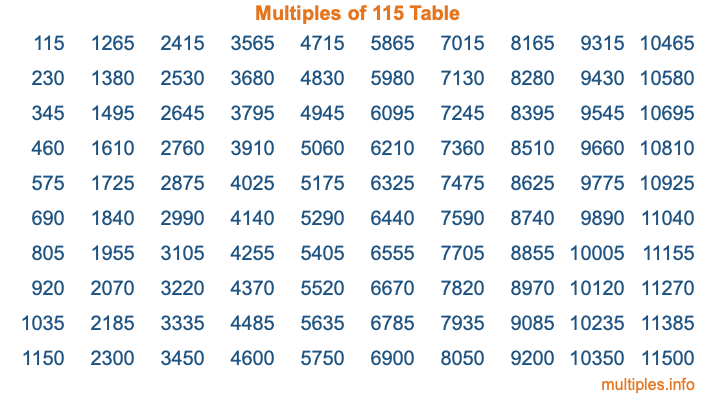Multiples of 115Welcome to the Multiples of 115 page. Here we will first teach you everything you will ever need to know about the multiples of 115, and then give you a study guide summary of everything we taught you to make sure you remember it all. Use this page to look up facts and learn information about the multiples of 115. This page will make you a multiples of one hundred fifteen expert!

Definition of Multiples of 115
Multiples of 115 are all the numbers that when divided by 115 equal an integer. Each of the multiples of 115 are called a multiple. A multiple of 115 is created by multiplying 115 by an integer.

Therefore, to create a list of multiples of 115, you start with 1 multiplied by 115, then 2 multiplied by 115, then 3 multiplied by 115, and so on for as long as you want. Thus, the list of the first five multiples of 115 is 115, 230, 345, 460, and 575. To see a larger list of multiples of 115, see the printable image of Multiples of 115 further down on this page. We also have a category where you can choose any nth multiple of 115.

Multiples of 115 Checker
The Multiples of 115 Checker below checks to see if any number of your choice is a multiple of 115. In other words, it checks to see if there is any number (integer) that when multiplied by 115 will equal your number. To do that, we divide your number by 115. If the the quotient is an integer, then your number is a multiple of 115.

Is  a multiple of 115?

Least Common Multiple of 115 and ...
A Least Common Multiple (LCM) is the lowest multiple that two or more numbers have in common. This is also called the smallest common multiple or lowest common multiple and is useful to know when you are adding our subtracting fractions. Enter one or more numbers below (115 is already entered) to find the LCM.

Check out our LCM Calculator if you need more details about the Least Common Multiple or if you need the LCM for different numbers for adding and subtraction fractions.

nth Multiple of 115
As we stated above, 115 is the first multiple of 115, 230 is the second multiple of 115, 345 is the third multiple of 115, and so on. Enter a number below to find the nth multiple of 115.

th multiple of 115

Multiples of 115 vs Factors of 115
115 is a multiple of 115 and a factor of 115, but that is where the similarities end. All postive multiples of 115 are 115 or greater than 115. All positive factors of 115 are 115 or less than 115.

Below is the beginning list of multiples of 115 and the factors of 115 so you can compare:

Multiples of 115: 115, 230, 345, 460, 575, etc.

Factors of 115: 1, 5, 23, 115

As you can see, the multiples of 115 are all the numbers that you can divide by 115 to get a whole number. The factors of 115, on the other hand, are all the whole numbers that you can multiply by another whole number to get 115.

It's also interesting to note that if a number (x) is a factor of 115, then 115 will also be a multiple of that number (x).

Multiples of 115 vs Divisors of 115
The divisors of 115 are all the integers that 115 can be divided by evenly. Below is a list of the divisors of 115.

Divisors of 115: 1, 5, 23, 115

The interesting thing to note here is that if you take any multiple of 115 and divide it by a divisor of 115, you will see that the quotient is an integer.

Multiples of 115 Table
Below is an image of the first 100 multiples of 115 in a table. The table is in chronological order, column by column. The first column has the first ten multiples of 115, the second column has the next ten multiples of 115, and so on.The Multiples of 115 Table is also referred to as the 115 Times Table or Times Table of 115. You are welcome to print out our table for your studies.

Negative Multiples of 115
Although not often discussed or needed in math, it is worth mentioning that you can make a list of negative multiples of 115 by multiplying 115 by -1, then by -2, then by -3, and so on, to get the following list of negative multiples of 115:

-115, -230, -345, -460, -575, etc.

Multiples of 115 Summary
Below is a summary of important Multiples of 115 facts that we have discussed on this page. To retain the knowledge on this page, we recommend that you read through the summary and explain to yourself or a study partner why they hold true.

There are an infinite number of multiples of 115.

A multiple of 115 divided by 115 will equal a whole number.

115 divided by a factor of 115 equals a divisor of 115.

The nth multiple of 115 is n times 115.

The largest factor of 115 is equal to the first positive multiple of 115.

115 is a multiple of every factor of 115.

115 is a multiple of 115.

A multiple of 115 divided by a divisor of 115 equals an integer.

115 divided by a divisor of 115 equals a factor of 115.

Any integer times 115 will equal a multiple of 115.

Multiples of a Number
Here you can get the multiples of another number, all with the same attention to detail as we did for multiples of 115 on this page.

Multiples of
Multiples of 116
Did you find our page about multiples of one hundred fifteen educational? Do you want more knowledge? Check out the multiples of the next number on our list!